NCERT Solutions for Maths Class 12 Exercise 7.3

# NCERT Solutions for Maths Class 12 Exercise 7.3

## NCERT Solutions for Maths Class 12 Exercise 7.3

Hello Students! Welcome to maths-formula.com. In this post, you will find the complete NCERT Solutions for Maths Class 12 Exercise 7.3.

You can download the PDF of NCERT Books Maths Chapter 7 for your easy reference while studying NCERT Solutions for Maths Class 12 Exercise 7.3.

Class 12th is a very crucial stage of your student’s life, since you take all important decisions about your career on this stage. Mathematics plays a vital role to take decision for your career because if you are good in mathematics, you can choose engineering and technology field as your career.

NCERT Solutions for Maths Class 12 Exercise 7.3 helps you to solve each and every problem with step by step explanation which makes you strong in mathematics.

All the schools affiliated with CBSE, follow the NCERT books for all subjects. You can check your syllabus from NCERT Syllabus for Mathematics Class 12.

NCERT Solutions for Maths Class 12 Exercise 7.3 are prepared by the experienced teachers of CBSE board. If you are preparing for JEE Mains and NEET level exams, then it will definitely make your foundation strong.

If you want to recall All Maths Formulas for Class 12, you can find it by clicking this link.

If you want to recall All Maths Formulas for Class 11, you can find it by clicking this link.

NCERT Solutions for Maths Class 12 Exercise 7.1

NCERT Solutions for Maths Class 12 Exercise 7.2

NCERT Solutions for Maths Class 12 Exercise 7.4

NCERT Solutions for Maths Class 12 Exercise 7.5

NCERT Solutions for Maths Class 12 Exercise 7.6

NCERT Solutions for Maths Class 12 Exercise 7.7

NCERT Solutions for Maths Class 12 Exercise 7.8

NCERT Solutions for Maths Class 12 Exercise 7.9

NCERT Solutions for Maths Class 12 Exercise 7.10

NCERT Solutions for Maths Class 12 Exercise 7.11

## NCERT Solutions for Maths Class 12 Exercise 7.3

Find the integrals of the functions in Exercises 1 to 22.

Maths Class 12 Ex 7.3 Question 1.

sin² (2x + 5)

Solution:

∫ sin² (2x + 5) dx = ½ ∫ [1 – cos 2(2x + 5)] dx
½ ∫ [1 – cos (4x + 10)] dx
=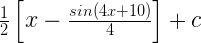Maths Class 12 Ex 7.3 Question 2.

sin 3x cos 4x

Solution:

∫ sin 3x cos 4x = ½ ∫ [sin (3x + 4x) + cos (3x – 4x)] dx
= ½ ∫ [sin 7x + sin (–x)] dx
= ½ ∫ sin 7x dx – ½ ∫ sin x dx
=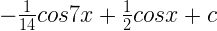Maths Class 12 Ex 7.3 Question 3.

cos 2x cos 4x cos 6x dx

Solution:

∫ cos 2x cos 4x cos 6x dx

= ½ ∫ (2 cos 2x cos 4x) cos 6x dx

= ½ ∫ (cos 6x + cos 2x) cos 6x dx

= ¼ ∫ (2 cos2 6x + 2 cos 2x cos 6x) dx

Maths Class 12 Ex 7.3 Question 4.

sin3 (2x + 1) dx

Solution:

∫ sin3 (2x + 1) dx = ¼ ∫ [3 sin (2x + 1) – sin 3(2x + 1)] dx

Maths Class 12 Ex 7.3 Question 5.

sin3 x cos3 x

Solution:

Maths Class 12 Ex 7.3 Question 6.

sin x sin 2x sin 3x

Solution:

∫ sin x sin 2x sin 3x dx = ½ ∫ (2 sin x sin 2x) sin 3x dx
½ ∫ (cos x – cos 3x) sin 3x dx

= ¼ ∫ (2 sin 3x cos x – 2 sin 3x cos 3x) dx
= ¼ ∫ (sin 4x + sin 2x – sin 6x)dx
=Maths Class 12 Ex 7.3 Question 7.

sin 4x sin 8x

Solution:

∫ sin 4x sin 8x dx = ½ ∫ 2 sin 4x sin 8x dx

½ ∫ (cos 4x – cos 12x) dx
=Maths Class 12 Ex 7.3 Question 8.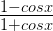Solution:

Maths Class 12 Ex 7.3 Question 9.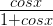Maths Class 12 Ex 7.3 Question 10.

sin4 x dx

Solution:

Maths Class 12 Ex 7.3 Question 11.

cos4 2x

Solution:

Maths Class 12 Ex 7.3 Question 12.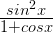Solution:

Maths Class 12 Ex 7.3 Question 13.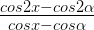Solution:

Maths Class 12 Ex 7.3 Question 14.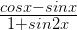Solution:

Maths Class 12 Ex 7.3 Question 15.

tan3 2x sec 2x

Solution:
Let I = ∫ tan3 2x sec 2x = ∫ (sec2 2x – 1) sec 2x tan 2x dx

= ∫ tan2 2x sec 2x tan 2x dx

Putting sec 2x = t, then, 2 sec 2x tan 2x dx = dt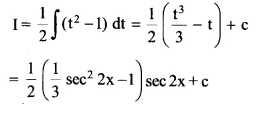Maths Class 12 Ex 7.3 Question 16.

tan4 x

Solution:

Let I = ∫ tan4 x dx = ∫ tan2 x tan2 x dx
= ∫ tan2 x (sec² x – 1) dx

= ∫ tan2 x sec² x dx – ∫ (sec² x – 1) dx

= ∫ tan2 x sec² x dx – ∫ (sec² x + ∫ 1 dx     … (i)

Putting tan x = t, then, sec² x dx = dt

From equation (i), we get

I = ∫ t2 dt – tan x + x + c

= t3/3 – tan x + x + c

= 1/3 tan3 x – tan x + x + c

Maths Class 12 Ex 7.3 Question 17.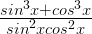Solution:

Maths Class 12 Ex 7.3 Question 18.Solution:

Maths Class 12 Ex 7.3 Question 19.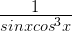Solution:

Maths Class 12 Ex 7.3 Question 20.Solution:

Maths Class 12 Ex 7.3 Question 21.

sin-1 (cos x)

Solution:

Maths Class 12 Ex 7.3 Question 22.Solution:

Maths Class 12 Ex 7.3 Question 23.(A) tan x + cot x + c
(B) tan x + cosec x + c
(C) –tan x + cot x + c
(D) tan x + sec x + c

Solution:

Maths Class 12 Ex 7.3 Question 24.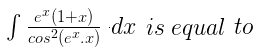(A) –cot (exx) + c
(B) tan (xex) + c
(C) tan (ex) + c
(D) cot (ex) + c

Solution: